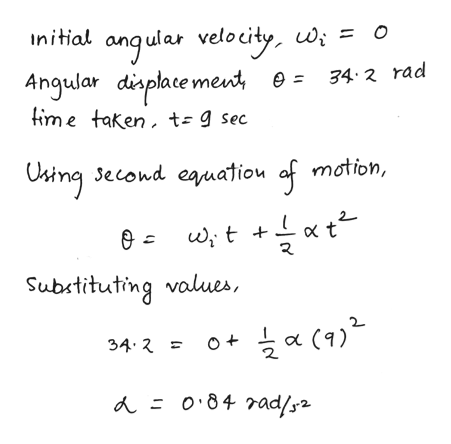# Starting from rest, a disk rotates about its central axis with constant angular acceleration. In 9.00 s, it rotates 34.2 rad. During that time, what are the magnitudes of (a) the angular acceleration (b) What is the instantaneous angular velocity of the disk at the end of the 9.00 s? (c) With the angular acceleration unchanged, through what additional angle (rad) will the disk turn during the next 9.00 s?

Question

Starting from rest, a disk rotates about its central axis with constant angular acceleration. In 9.00 s, it rotates 34.2 rad. During that time, what are the magnitudes of (a) the angular acceleration (b) What is the instantaneous angular velocity of the disk at the end of the 9.00 s? (c) With the angular acceleration unchanged, through what additional angle (rad) will the disk turn during the next 9.00 s?

check_circleExpert Solution
Step 1

Given:

In 9 seconds a disc rotates 34.2 radians.

Step 2

Calculating the angular acceleration:help_outlineImage Transcriptioncloseangular velocity wi Angular displace ment e= 34 2 rad lime taken, t- 9 sec O Ostng Jecond eauatiou motion, of ewt t Substituting values, 34 2 O (9) A = o84 rad/2 fullscreen
Step 3

Calculating the instantaneous angular velocity at 9 seconds...

### Want to see the full answer?

See Solution

#### Want to see this answer and more?

Solutions are written by subject experts who are available 24/7. Questions are typically answered within 1 hour*

See Solution
*Response times may vary by subject and question
Tagged in

### Angular Motion